# Quantitative Aptitude Quiz for Prelims Exams- SBI & IBPS 2020- 15th December

Directions (1-5): What will come in the place of question (?) mark in following number series.

Q1. 567, 571, ? , 623, 687, 787
(a) 615
(b) 599
(c) 587
(d) 601
(e) 593

Q2. 167, 118, 76, 42, ?, -4
(a) 17
(b) 14
(c) 18
(d) 16
(e) 25

Q3. ?, 120, 134, 160, 204, 272
(a) 112
(b) 104
(c) 106
(d) 114
(e) 100

Q4. 5, ? , 2067, 2411, 2537, 2565
(a) 1337
(b) 1327
(c) 1317
(d) 1307
(e) 1347

Q5. 427, ? , 366, 73.2, 292.8, 97.6
(a) 51
(b) 41
(c) 61
(d) 71
(e) 31

Directions (6-10): What will come in the place of question (?) mark in following questions.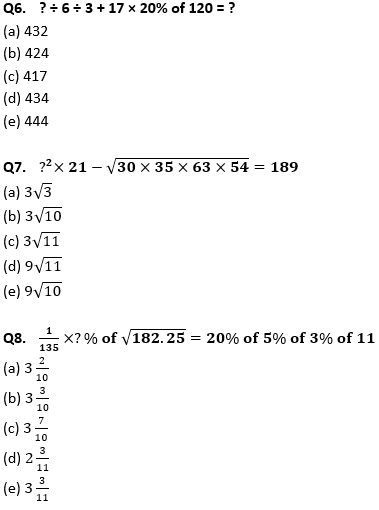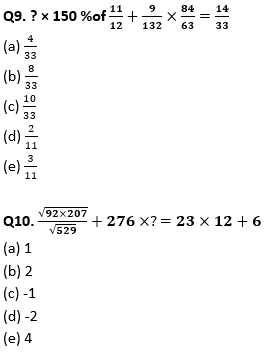Directions (11-15): In the following questions two quantities are given for each question. Compare the numeric value of both the quantities and answers accordingly.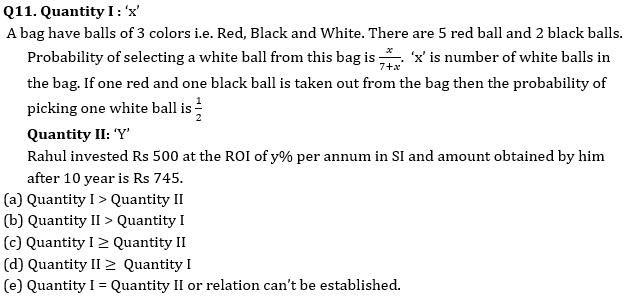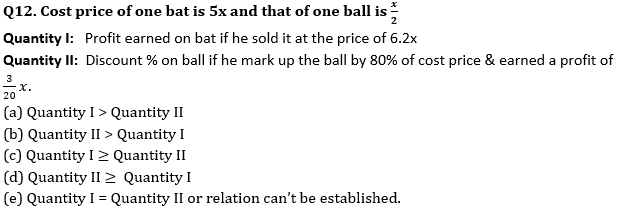Q13.Quantity I : curved surface area of a cone.
If the base radius of cone is 8 cm & height is 25% less than its radius.
Quantity II: Curved surface area of a cylinder.
If maximum volume of cylinder is 200π and its height is 60% more than its radius.
(a) Quantity I > Quantity II
(b) Quantity II > Quantity I
(c) Quantity I ≥ Quantity II
(d) Quantity II ≥ Quantity I
(e) Quantity I = Quantity II or relation can’t be established.

Q14.Quantity I: Number of days taken by A to complete the work.
If A, B & C working all together can complete the work in 4 days & B & C together takes 6 days to complete it. C is 50% more efficient than B.
Quantity II: Number of hours taken by most efficient pipe to fill the tank.
Three pipes P, Q and R working alternatively in the cycle P→ Q→ R for 1 hour each, can fill tank in 15 hours. A pipe P alone will the take 20 hours, & ratio of time taken by pipe Q to R is 3 : 2 to fill the tank.
(a) Quantity I > Quantity II
(b) Quantity II > Quantity I
(c) Quantity I ≥ Quantity II
(d) Quantity II ≥ Quantity I
(e) Quantity I = Quantity II or relation can’t be established.

Q15. Average age of A, B and C is 33 year. Ratio of age of B to C is 11 : 13 and age of A is 10% less than the average age of A and B.
Quantity I→ Age of B
Quantity II→ Average of A and C
(a) Quantity I > Quantity II
(b) Quantity II > Quantity I
(c) Quantity I ≥ Quantity II
(d) Quantity II ≥ Quantity I
(e) Quantity I = Quantity II or relation can’t be established.

Practice More Questions of Quantitative Aptitude for Competitive Exams:

###### Prelims Quiz Study Plan For SBI & IBPS Exams 2020

Solutions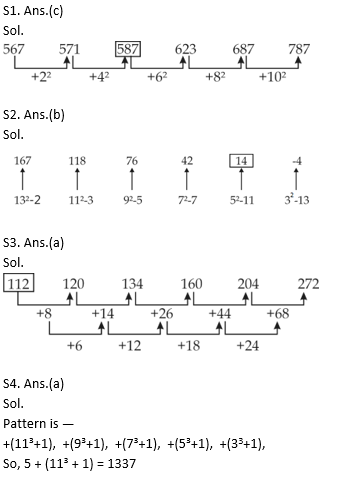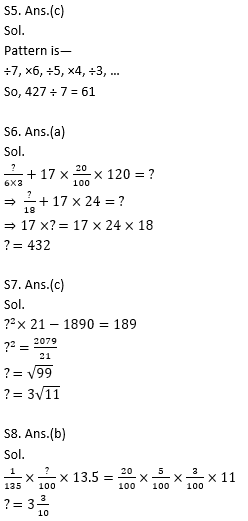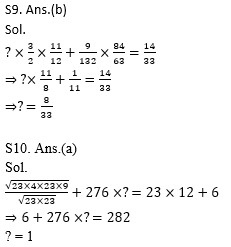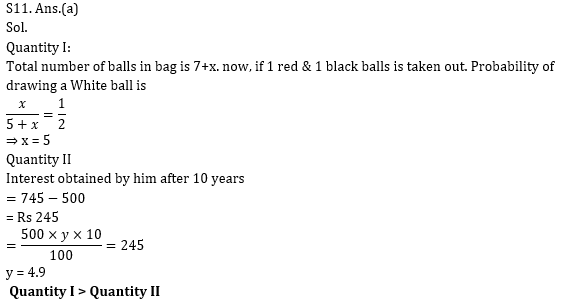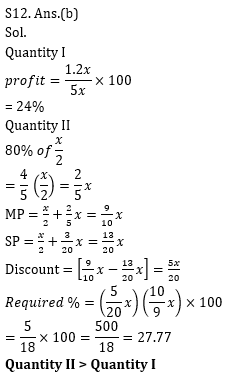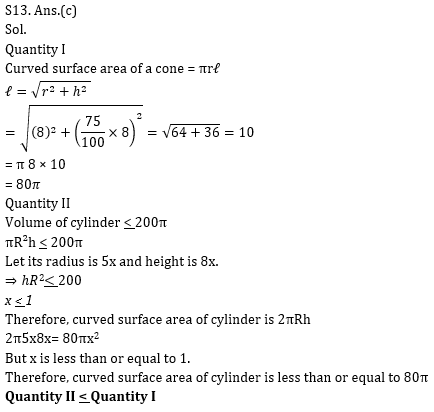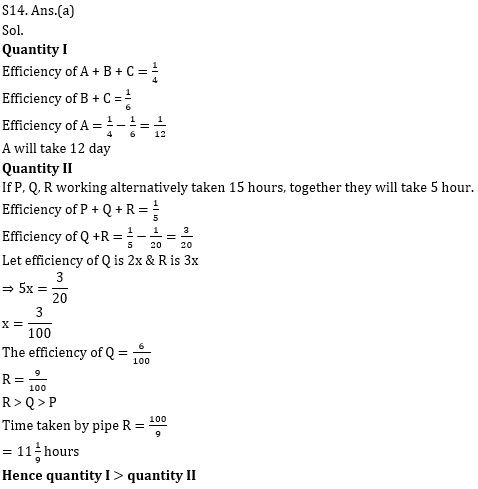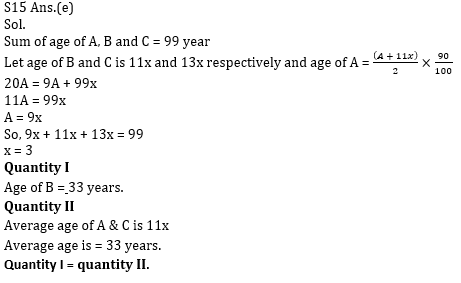Practice with Crash Course and Online Test Series for IBPS Clerk Prelims: